# The survRatio package

## Estimating, Comparing and Visualising Time to Event Data

The survRatio is an R package which provides numerical and graphical summaries for time to event data. In this release functions are provided to estimate and compare estimated survivor functions ($$S_1(t),S_2(t)$$), the ratio of survivor functions ($$\frac{S_1(t)}{S_2(t)}$$) and the difference of survivor functions ($$S_1(t)-S_2(t)$$) in independent and paired time to event problems. Time intervals where the survival prospects may differ are identified using pointwise confidence bands .

### Example 1: Independent survival data

The lung cancer dataset from the survival package includes the survival time (in days) of male and female patients with advanced lung cancer from the North Central Cancer Treatment Group. These data are used to illustrate the comparison of two (independent) survivor functions.

The drsurv function takes the survival time, censoring indicator and the factor level as inputs and returns the Kaplain-Meier estimated survivor functions with their corresponding confidence intervals. A drsurv object is created which contains the estimates of the survival ratio, the survival difference and pointwise (bootstrap) confidence bands.

fit1 <- drsurv(time = lung.time, status = lung.cens, factor = gender)
ls(fit1)

##  "clevel" "levels" "nboot"  "paired" "pvalue" "surv"


The drsurv object also includes the assigned confidence level, the factor levels, the number of bootstrap replicates and the p-value for the test of equality of survivr functions.

head(fit1$surv)  time nrisk1 nrisk2 surv1 l.surv1 u.surv1 surv2 l.surv2 u.surv2 diff lower.diff upper.diff ratio lower.ratio upper.ratio 5.0 138 90 1.000 0.954 1.000 0.989 0.967 1 0.0111 0.0000 0.0353 1.010 1.000 1.040 15.3 132 90 0.949 0.913 0.987 0.989 0.967 1 -0.0396 -0.0853 0.0010 0.960 0.914 1.000 25.5 132 90 0.949 0.913 0.987 0.989 0.967 1 -0.0396 -0.0853 0.0010 0.960 0.914 1.000 35.8 129 90 0.928 0.885 0.972 0.989 0.967 1 -0.0614 -0.1120 -0.0130 0.938 0.888 0.987 46.1 129 90 0.928 0.885 0.972 0.989 0.967 1 -0.0614 -0.1120 -0.0130 0.938 0.888 0.987 56.4 126 90 0.906 0.858 0.956 0.989 0.967 1 -0.0831 -0.1370 -0.0330 0.916 0.862 0.967 A plot of the survival ratio ($$\frac{\hat{S_1}(t)}{\hat{S_2}(t)}$$) with pointwise confidence bands can be generated using the ggsurv function: ggsurv(fit1) + ylab("Estimated Survival Ratio") + xlab("Follow Up Time (days)")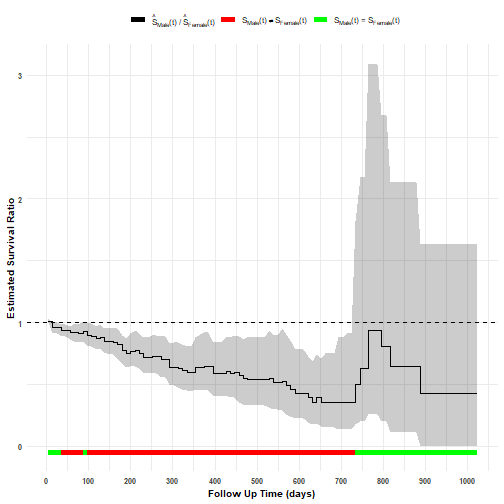The default, plot displays the estimated survival ratio and 95% (pointwise) confidence interval. In addition, a reference line is drawn where the ratio is equal to 1 (to represent 'no difference' in the survival ratio) and time intervals coloured by whether or not the (pointwise) confidence bands include the reference. For example, there appears to be convincing evidence of a difference in survival prospects favoring females up to approximately 730 days. The plot is generated as a ggplot object and allowing changes to axis labels, themes etc: ggsurv(fit1) + ylab("Estimated Survival Ratio") + xlab("Follow Up Time (days)") + theme_bw()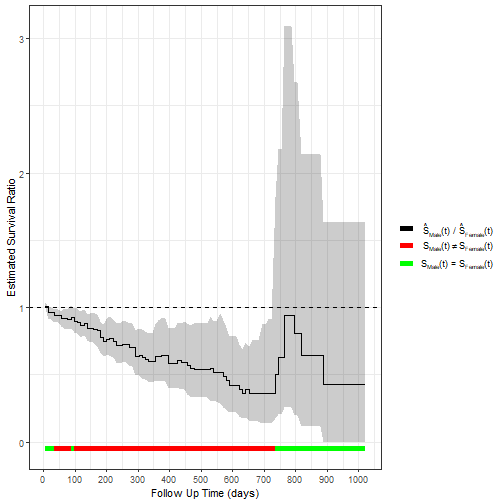A plot of the survival difference ($$\hat{S_1}(t)-\hat{S_2}(t)$$) with pointwise confidence bands can be generated by setting statistics = "diff" as follows: ggsurv(fit1, statistics = "diff") + ylab("Estimated Difference in Survival") + xlab("Follow Up Time (days)")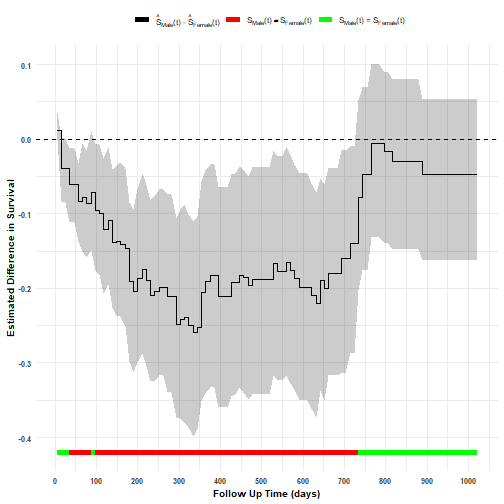The ggsurv function has the option to include a table of the number of subjects at risk over time: ggsurv(fit1, statistics = "diff", palette = c("blue"), xlab = "Time (days)", ylab = "Estimated Difference in Survival", theme = "gray", table = TRUE)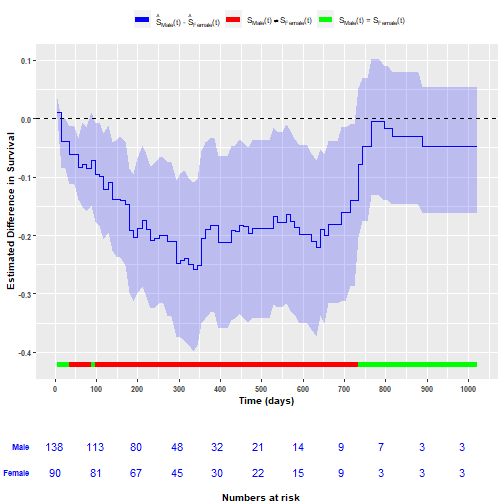A plot of the Kaplan-Meir estimated survivor functions ($$\hat{S_1}(t)$$ and $$\hat{S_2}(t)$$), accompanied with their confidence intervals and p-value from a log-rank test, can be generated by setting statistics = "surv": ggsurv(fit1, statistics = "surv", p.value = TRUE) + ylab("Estimated Survival") + xlab("Follow Up Time (days)")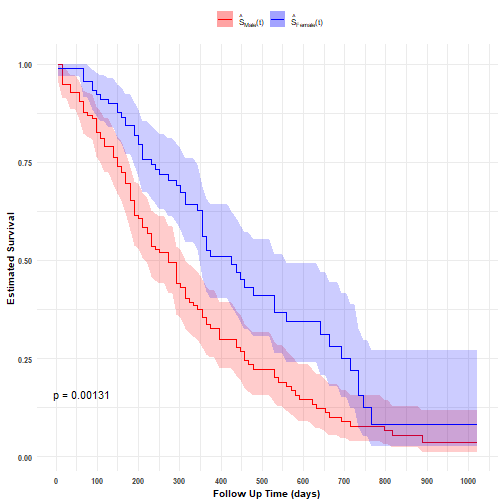If required, the ggsurv function can provided a grid of the three plots by setting statistics = "all". ggsurv(fit1, statistics = "all", table = TRUE)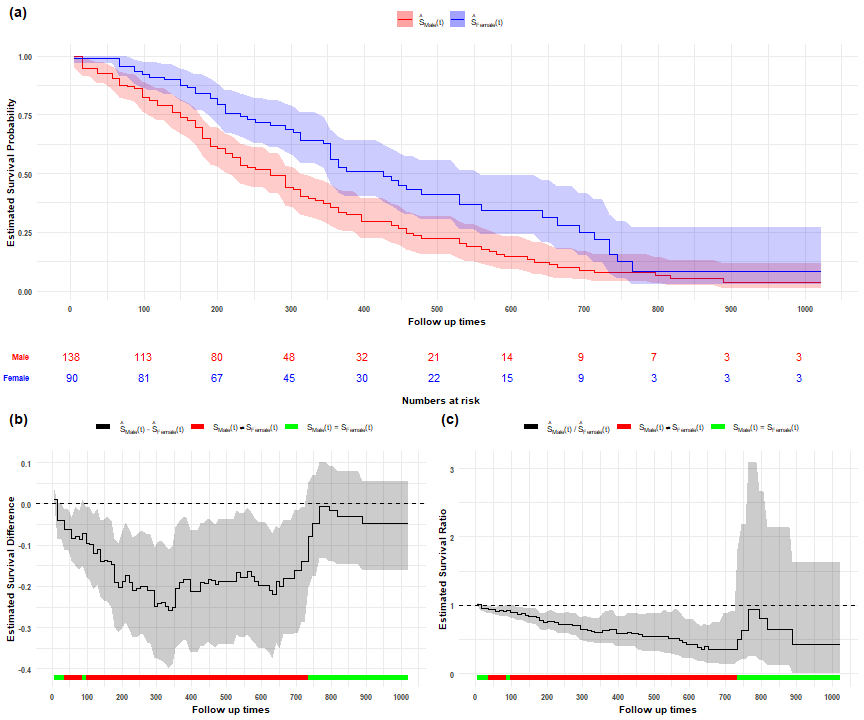### Example 2: Paired survival data The diabetic retinopathy dataset from the survival package is used to illustrate the comparison of survival prospects for a paired design. The dataset includes time to loss of vision (in months) after laser coagulation as a treatment to delay diabetic retinopathy. Each patient has two observations in the data set as one eye was randomised to receive laser treatment with the other eye receiving no treatment. The drsurv function can be used for paired survival design to estimate the (marginal) survivor functions for each treatment, pairwise differences and ratios (with corresponding pointwise confidence bands based on a permutation test) by setting paired=TRUE and providing a variable to identify each pair. fit2 <- drsurv(ret.time, ret.status, ret.treatment, paired = TRUE, id = ret.id) head(fit2$surv)

time nrisk1 nrisk2 surv1 l.surv1 u.surv1 surv2 l.surv2 u.surv2 diff lower.diff upper.diff ratio lower.ratio upper.ratio
0.30 197 197 0.995 0.985 1.000 1.000 0.985 1.000 -0.00508 -0.0152 0.00e+00 0.995 0.985 1.000
1.05 195 197 0.985 0.968 1.000 1.000 0.985 1.000 -0.01520 -0.0355 0.00e+00 0.985 0.964 1.000
1.81 184 192 0.939 0.906 0.973 0.979 0.960 1.000 -0.04080 -0.0814 -5.08e-03 0.958 0.918 0.995
2.56 182 190 0.928 0.893 0.965 0.969 0.945 0.994 -0.04080 -0.0865 7.37e-05 0.958 0.912 1.000
3.32 178 189 0.913 0.874 0.953 0.964 0.938 0.991 -0.05110 -0.0972 -5.13e-03 0.947 0.900 0.995
4.07 177 189 0.908 0.868 0.949 0.964 0.938 0.991 -0.05630 -0.1020 -1.03e-02 0.942 0.895 0.989

A plot of the survival ratio can be generated while incorporating ggplot commands as required:

p1 <- ggsurv(fit2, statistics = "ratio")
p1 + labs(title = "Estimated Survival Ratio for Time to Loss of Vision after Laser Coagulation",
subtitle = "Ratio = (Control / Treated)",
y = "Estimated Survival Ratio",
x = "Follow Up (Months)")  +
theme(legend.position="bottom",
legend.background = element_rect(fill="lightblue", linetype="solid"))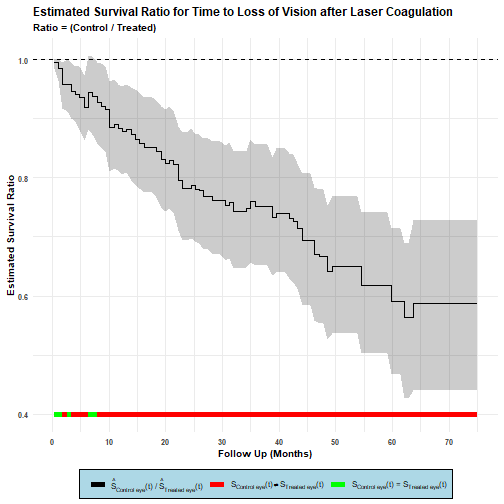A (annotated) plot of the survival difference is as follows:

p1 <- ggsurv(fit2, statistics = "diff")
p1 + labs(title = "Estimated Survival Difference for Time to Loss of Vision after Laser Coagulation",
subtitle = "Difference = (Control - Treated)",
y = "Estimated Survival Difference",
x = "Follow Up (Months)")  +
theme(legend.position="bottom",
legend.background = element_rect(fill="white", linetype="solid"))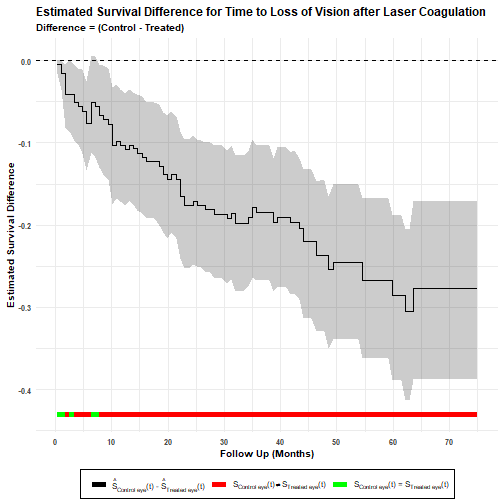## Reference

 Newell, J., Kay, J. W., & Aitchison, T. C. (2006). Survival ratio plots with permutation envelopes in survival data problems. Computers in biology and medicine, 36(5), 526-541.